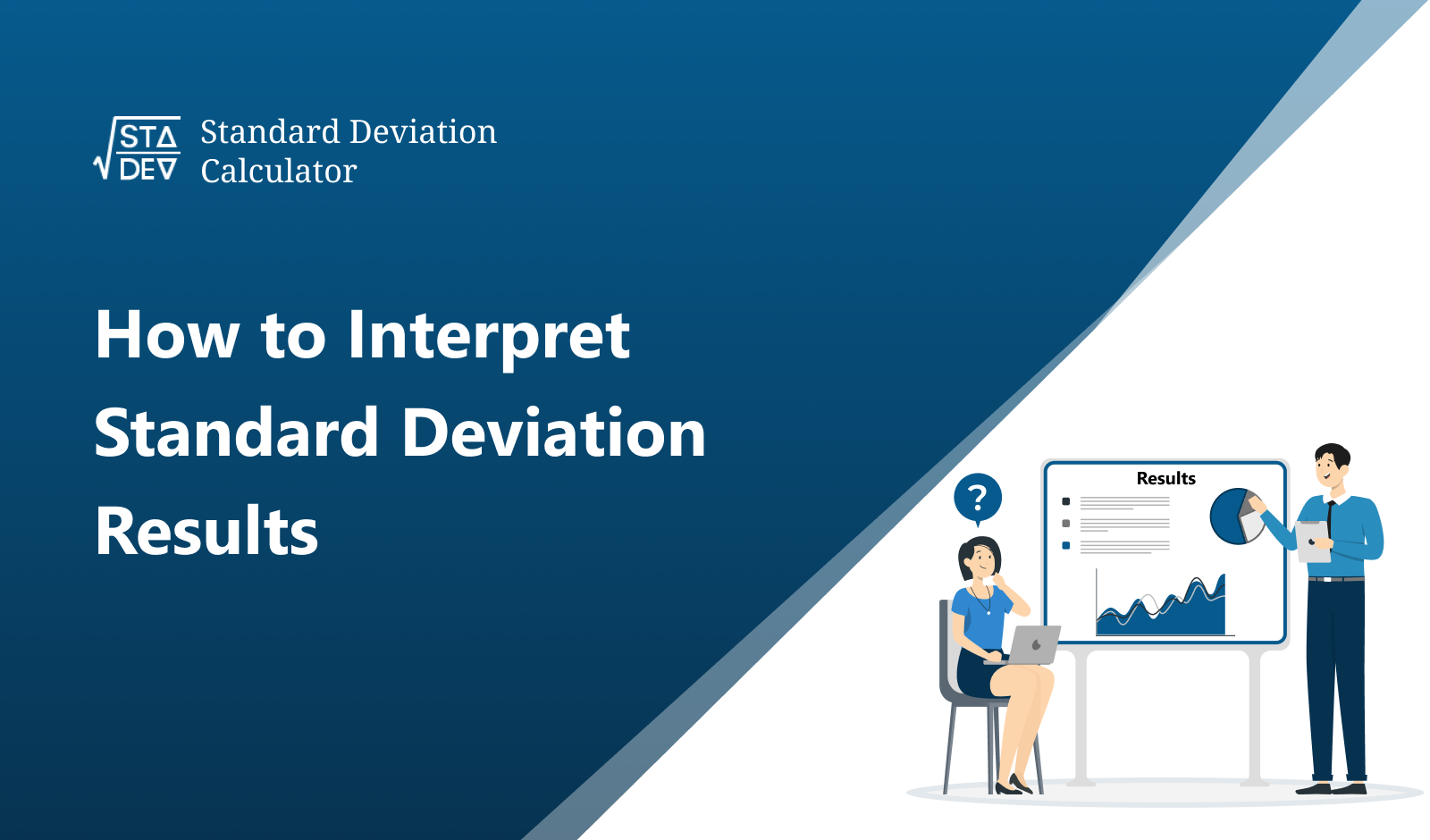Jul 4, 2023
How to Interpret Standard Deviation ResultsIn the realm of statistical analysis, Standard Deviation is a term that often emerges. It is a measure used in statistics that quantifies the amount of dispersion or variation in a set of values. But how does one interpret the results derived from calculating the standard deviation?

If you're keen to unravel the mysteries of standard deviation, then you've come to the right place. This article is dedicated to helping you interpret standard deviation results with confidence and clarity.

## What is Standard Deviation?

Before delving into the interpretation, let's brush up on what standard deviation is. Standard deviation is a statistic that measures the dispersion of a dataset relative to its mean. The standard deviation is calculated as the square root of variance by determining each data point's deviation relative to the mean.

If the data points are further from the mean, there is a higher deviation within the data set; thus, the more spread out the data, the higher the standard deviation.

## Understanding the Importance of Standard Deviation:

Standard Deviation is a crucial statistical tool because it allows us to comprehend the degree of variation in a dataset. It provides insights into how much variation or "dispersion" exists from the average (mean), or expected value.

A low standard deviation signifies that the values tend to be close to the mean, whereas a high standard deviation indicates that the values are spread out over a wider range.

## How to Calculate Standard Deviation?

The standard deviation can be calculated using various tools and techniques. These range from manual calculations to sophisticated statistical software. However, one of the simplest and most convenient methods is using an online tool, such as the Standard Deviation Calculator.

This user-friendly platform offers an effortless way to compute the standard deviation for any given data set.

## Interpreting Standard Deviation Results:

Interpreting the results of standard deviation involves understanding its relationship with the mean and the overall data set.

### Low Standard Deviation:

A low standard deviation indicates that the data points are generally close to the mean or the expected value. This implies that there is less variability in the data set, and the values are relatively consistent.

### High Standard Deviation:

In contrast, a high standard deviation indicates that data points are spread out over a large range of values. This spread signifies a higher level of variability or volatility within the dataset.

## Standard Deviation in Real-World Scenarios:

The interpretation of standard deviation becomes much more relatable when applied to real-world scenarios. For instance, in finance, a high standard deviation of stock returns would imply higher volatility and, thus, a riskier investment. In research studies, a high standard deviation might reflect a larger spread of data, which could influence the study's reliability and validity.

## Conclusion

Understanding and interpreting standard deviation results is a skill that proves valuable across multiple disciplines, from finance to scientific research. Armed with the Standard Deviation Calculator and the knowledge from this article, you're well on your way to interpreting standard deviation results with ease.

Remember, a low standard deviation signifies consistency, while a high standard deviation denotes variability. Here's to making confident, data-driven decisions!